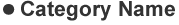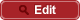• HOME
• RIKEN-QHP
• Measurements of the absolute branching fractions of $B^+ \to X_{c\bar{c}} K^+$ and $B^+ \to \bar{D}^{(\ast)0} \pi^+$ at Belle

##PrePrint No. RIKEN-QHP-349 Y. Kato et al., (including M. Takizawa) (Belle Collaboration) Measurements of the absolute branching fractions of $B^+ \to X_{c\bar{c}} K^+$ and $B^+ \to \bar{D}^{(\ast)0} \pi^+$ at Belle arXiv: 1709.06108  (org) 2017.09